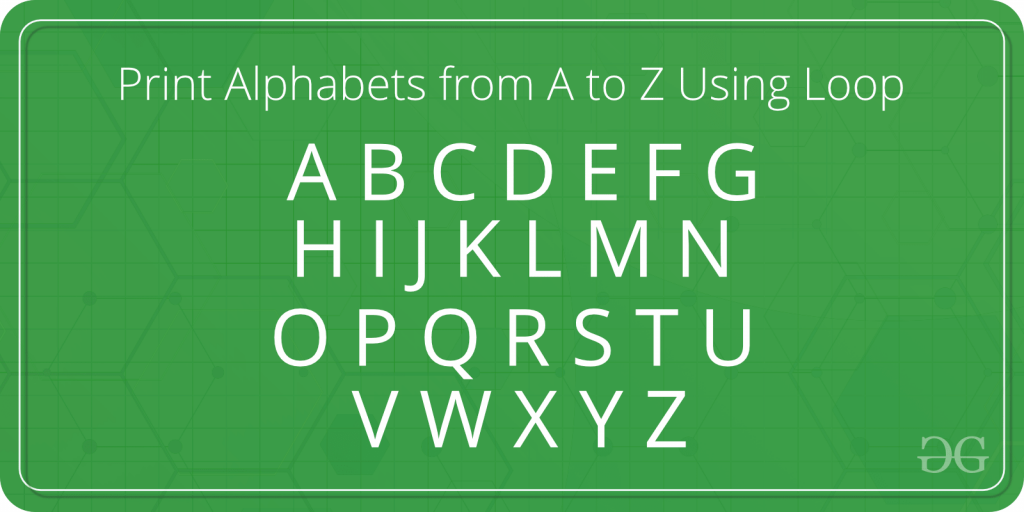Open in App
Not now

# JavaScript program to print Alphabets from A to Z using Loop

• Last Updated : 04 Nov, 2022

Our task is to print the alphabet from A to Z using loops. In this article we will mainly focus on the following programs and their logic:

• Using for loop
• Using the while loop
• Using a do-while loop#### Program to print (A to Z) and (a to z) using for loop:

In the below program:

• For loop is used to print the alphabets from A to Z. A loop variable is taken to do this of type ‘char’.
• The loop variable ‘i’ is initialized with the first alphabet ‘A’ and incremented by 1 on every iteration.
• In the loop, the character ‘i’ is printed as the alphabet.

Example: Below is the example that will print (A to Z) and (a to z) using for loop:

## Javascript

 ``

Output:

```Alphabets form (A-Z) are:
A B C D E F G H I J K L M N O P Q R S T U V W X Y Z
Alphabets from (a-z) are:
a b c d e f g h i j k l m n o p q r s t u v w x y z
```

#### Program to print (A to Z) and (a to z) using while loop:

In the below program:

• While loop is used to print the alphabets from A to Z. A loop variable is taken to display of type ‘char’.
• The loop variable ‘i’ is initialized with the first alphabet ‘A’ and incremented by 1 on every iteration.
• In the loop, the character ‘i’ is printed as the alphabet.

Example: Below is the example that will print (A to Z) and (a to z) using for while loop:

## Javascript

 ``

Output:

```Alphabets form (A-Z) are:
A B C D E F G H I J K L M N O P Q R S T U V W X Y Z
Alphabets from (a-z) are:
a b c d e f g h i j k l m n o p q r s t u v w x y z
```

#### Program to print (A to Z) and (a to z) using do-while loop:

In the below program:

• The do-while loop is used to print the alphabet from A to Z. A loop variable is taken to display of type ‘char’.
• The loop variable ‘i’ is initialized with the first alphabet ‘A’ and incremented by 1 on every iteration.
• In the loop, the character ‘i’ is printed as the alphabet.

Example: Below is the example that will print (A to Z) and (a to z) using for do-while loop:

## Javascript

 ``

Output:

```Alphabets form (A-Z) are:
A B C D E F G H I J K L M N O P Q R S T U V W X Y Z
Alphabets from (a-z) are:
a b c d e f g h i j k l m n o p q r s t u v w x y z
```

My Personal Notes arrow_drop_up